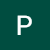# Hypothesis Testing

We often hear companies advertise dandruff shampoos that they claim are 100% effective, are these results realistically possible?

Hypothesis tests give a way of using samples to test whether statistical claims are likely to be true or not about a population.

A statistical measure for testing an assumption based on a population parameter. The technique for testing depends on the type of the data used and the cause for the analysis.

Hypothesis testing is used for situations where an assessment of the probable existence of an assumption or hypothesis can be ascertained with the help of sample data. …

# Decision Trees

An introduction to Decision trees — a Machine Learning algorithm used as a foundation for complex ensemble techniques.

Decision Tree is a supervised learning algorithm used for predictive modeling and solving CART — classification and regression problems. It generates a tree in the form of simple ‘if-then’ rules where each node represents a feature(attribute), each link(branch) represents a decision(rule) and each leaf represents an outcome(categorical or continuous value).

It is used as a foundation for many classical machine learning algorithms like Random Forest, Bagging, and Boosted Decision Trees.

Geometrically, the ‘if-then’ rules are axis parallel cuts forming rectangles in 2D…